# Line integration techniques

We test a few vertical-line-integration techniques

#include "line_integrate.h"
#include "utils.h"

scalar s[], S[];

int main() {
L0 = 10;
X0 = Y0 = -L0/2.;
init_grid (N);
S[top] = dirichlet (0.);

The source is a Gaussian blob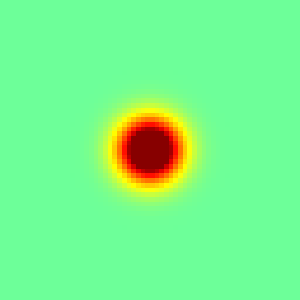foreach()
s[] = exp(-sq(x) - sq(y));
output_ppm (s, file = "s.png", n = 300);

## Option 1

Option 1 Works OK for this case.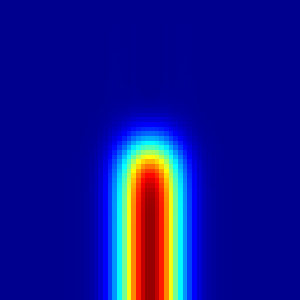Option 1

  integrate_dy (S, s);
output_ppm (S, file = "S1.png", n = 300, min = 0, max = sqrt(pi));

## Option 2

Option 2 seems OK with a modified tolerance.Option 2

  integrate_dn (S, s, tolerance = 1e-2);
output_ppm (S, file = "S2.png", n = 300, min = 0, max = sqrt(pi));

## Option 3

Option 3 seems OK with a modified tolerance.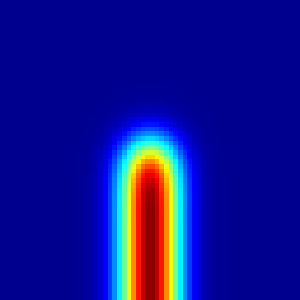Option 3

   integrate_1st (S, s, tolerance = 1e-2);
output_ppm (S, file = "S3.png", n = 300, min = 0, max = sqrt(pi));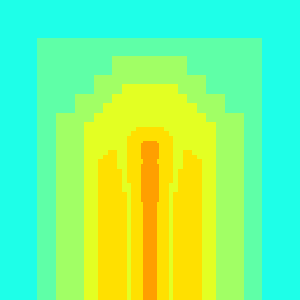Grid level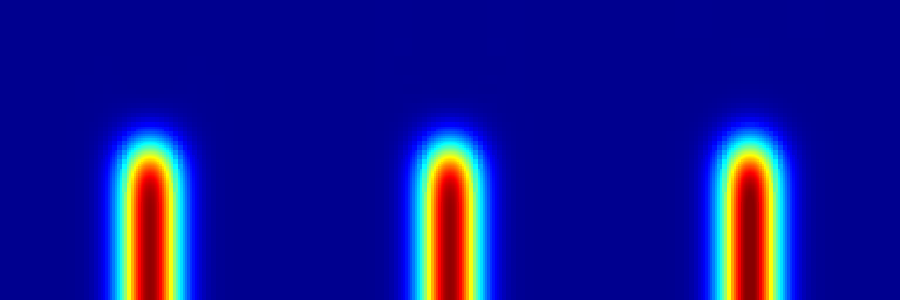Results for option 1, 2 and 3

   while (adapt_wavelet ({s, S}, (double[]){0.01, 0.01}, 8).nf);
scalar lev[];
foreach()
lev[] = level;
output_ppm (lev, file = "l.png", n = 300);
integrate_dy (S, s);
output_ppm (S, file = "S1a.png", n = 300, min = 0, max = sqrt(pi));
integrate_dn (S, s, tolerance = 1e-2);
output_ppm (S, file = "S2a.png", n = 300, min = 0, max = sqrt(pi));
integrate_1st (S, s, tolerance = 1e-2);
output_ppm (S, file = "S3a.png", n = 300, min = 0, max = sqrt(pi));
system ("convert S*a.png +append aS.png");

## Source at bottom boundary

Now the result from the previous integration is integrated.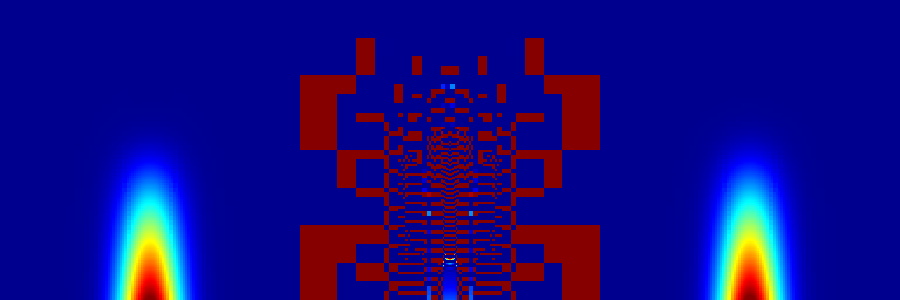Only option 2 is not able to do this

   boundary ({S});
s[bottom] = neumann ((S[] + S[0,-1])/2.);
s[top] = dirichlet (0);
foreach()
s[] = 0;
boundary ({s});
integrate_dy (s, S, tolerance = 1e-2);
output_ppm (s, file = "sintS1.png", n = 300, min = 0, max = L0/2*sqrt(pi));
foreach()
s[] = 0;
boundary ({s});
integrate_dn (s, S, tolerance = 1e-2);
output_ppm (s, file = "sintS2.png", n = 300, min = 0, max = L0/2*sqrt(pi));
foreach()
s[] = 0;
boundary ({s});
integrate_1st (s, S, tolerance = 1e-2);
output_ppm (s, file = "sintS3.png", n = 300, min = 0, max = L0/2*sqrt(pi));
system ("convert sintS*.png +append as.png");

## Conclusion

Option 3 is not a good idea, option 1 is.

}